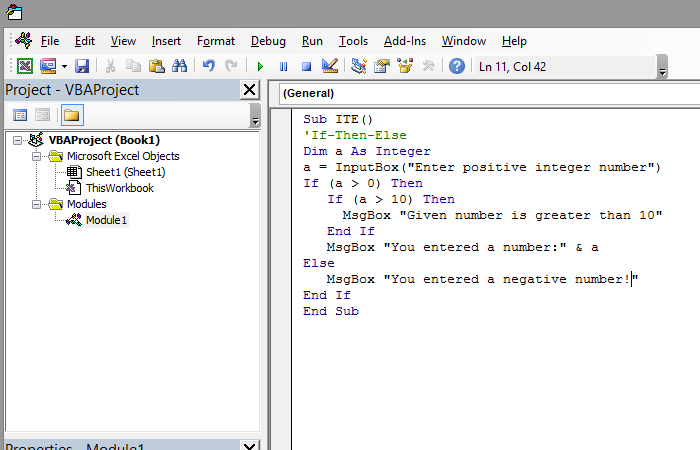Checking the logical condition

Checking the logical condition is done using the If-Then-Else structure. It starts with the command If, followed by a logical condition that is formed in the same way as in Excel. Next follows the command Then, aftwer which goes the part of the code to be executed if the condition is met. Optionally, Else command can be specified, followed by a part of the code that is executed if the condition is not met. The structure ends with an End If command, which is not necessary if there’s only one command after Then.

The syntax of this structure is:

If (<condition>) Then
[CODE IF THE CONDITION IS FULFILLED]
[Else]
[CODE IF THE CONDITION IS NOT COMPLETED]
[End If]

Let’s start with showing the simplest way of applying the If-Then structure.

Dim as As Integer
a = 10
If (a> 5) Then MsgBox “Set number is greater than 5”

The result of this program is the message that the number is greater than 5. If we wanted to get the message, otherwise we would write the following code:

Dim as As Integer
a = 10
If (a> 5) Then
MsgBox “Set number is greater than 5”
Else
MsgBox “Set number is less than 5”
End If

When we examine several conditions, it is possible to form the nested If-Then structure.

Dim as As Integer
a = InputBox (“Enter a positive integer number”)
If (a> 0) Then
If (a> 10) Then
MsgBox “Given number is greater than 10”
End If
MsgBox “You entered a number:” & a
Else
MsgBox “You entered a negative number!”
End If

The program we wrote first expects to enter a positive integer number. If it is greater than zero the second condition examines whether it is greater than ten and gives a message. Then, as a default, appears a message about a number you’ve entered. If the number entered is less than zero, the message about entering a negative number is displayed.